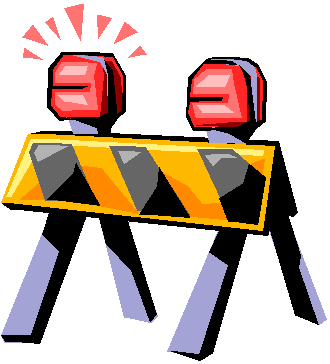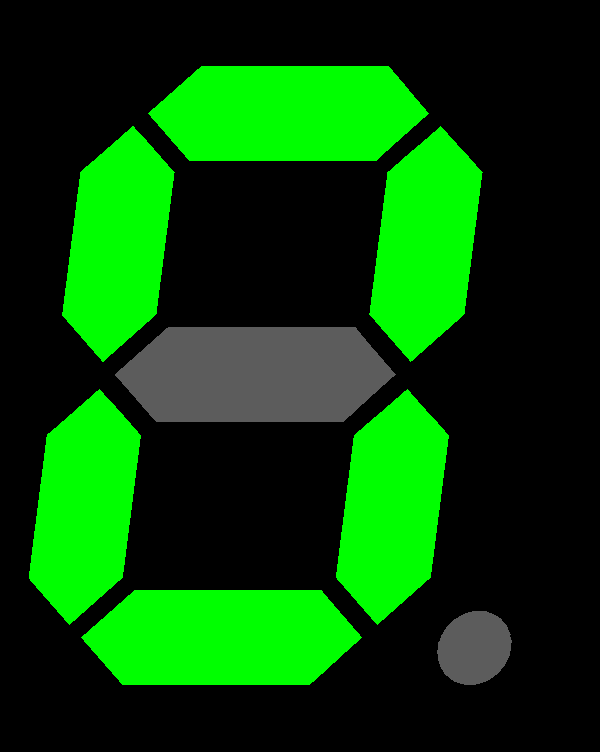Now that we have introduced adding we just cannot get enough and want more, more, more!! In this chapter it is all about the tricks and strategies that help us add. Here we will look at the strategies that most mathematicians use to add quickly. We model these strategies so that children can see them in action and introduce them so that children have a bigger catalog of strategies to use. Learning these strategies will allow the child to do more “mental math” or solve math sentences in her head instead of having to always count again from the beginning.

**Caution**There are a good number of kids that come to First Grade with some or all of these strategies already in their math catalog so these lessons can be a little frustrating. For the student that already uses a strategy that he feels comfortable with and has had success with to have to try a new strategy seems pointless. The point of these lessons is to expose children to all of the strategies so that everyone can find the one that works for them. All I ask is that for that particular lesson the child tries this strategy and be open to the idea. If the child goes back to a strategy that has worked for them then that it is fine .

The goal is for math success so … Here we go!!

1. Counting On and Adding in Any OrderUse the counting-on strategy when one number is 1. 2. or 3. Counting on with numbers that are higher than is not effective and often results in mistakes. In class we usually say “Put the bigger number in your head and count on” and then the students use their fingers to count on the other numbers.

Example- 3+5=

Put 5 in your head and then count on… 6, 7, 8 .

By learning to count on from the bigger number, the child is also learning that you can add numbers in any order.

Example- To practice this try the following:

Ask the child what 6+3 is and then after the answer immediately ask what 3+6 is. It is also helpful to model the math sentences with different objects for each number and write the sentences in colors. These techniques help the visual learner.

1.   Doubles and Doubles Plus 1Doubles come naturally to children. One of the first facts that they learn is 1+1=2.   There are a lot of connections to real life with doubles also, such as five fingers on one hand and five on the other is 10 fingers. If the children can learn the doubles then they can add those particular facts quickly and then try adding on to the double. In class, we have some rhymes that help to remember the doubles:

1+1=2             Little Bunny Foo Foo

2+2=4             Watch it pour!

3+3=6             I lose my teeth at 6

4+4=8             Spider’s on the gate

5+5=10          Tickle my abdomen

6+6=12          Ding-a-ling Do you hear the bell?

7+7=14          Two weeks. You know what I mean

8+8=16          Sweet Sixteen and never been kissed

9+9=18          Do you smell bacon?

10+10=20      You’re my little honey!

We will be going over these little rhymes often in class and it would help to practice them at home also.

Once the doubles facts are learned then the strategy of Doubles plus 1 can be used.

Example: 5+6 can be thought of as:

5 + (5+1)       Break apart the 6 then

(5+5) +1        Find the double then

10 +1              Add the doubles then

This is what is happening in the brain when one tries to figure out 5+6. We are modeling this so that everyone can understand the strategy.

2.   Making 10As was previously mentioned we LOVE the number 10 so it is very important to learn the ways to make 10. These are:

0+10=10

1+9=10

2+8=10

3+7=10

4+6=10

5+5=10

6+4=10

7+3=10

8+2=10

9+1=10

10+0=10

Knowing these facts is the same as knowing the doubles. By learning these facts then the child can make connections and find ten and then add on. 10 is definitely our friend!!

These are strategies are the beginning to understanding the tricks to adding. After practicing these strategies your child will understand that ADDING IS AWESOME!!

Activities for Chapter 3

1.     Using strategies to solve facts

Materials needed: 12 counters

Procedure:

1.       Hand the child 5 counters

2.       Place an additional counter in his hand and ask How can you find the total number of counters?

3.       Have the child tell the answer and explain how she solved it. Hopefully the child will have counted on, instead of recounting all of the counters.

4.       Repeat with the same 5 counters to start and then placing 2 more counters in her hand and then placing 3 counters in her hand.

2.     Sums of 10

Materials needed: 10 counters

Procedure:

1.Have the child line up items in a row and then take away any number of counters and hide them.

2.   A partner finds the missing number and explains how he figured out how many were missing.

3. Reverse the roles and observe the way that the child figures out how many are missing.